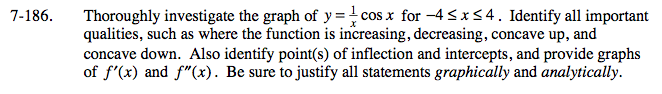### Home > CALC > Chapter 7 > Lesson 7.4.2 > Problem7-186

7-186.

Thoroughly investigate the graph of y =cos x for −4 ≤ x ≤ 4. Identify all important qualities, such as where the function is increasing, decreasing, concave up, and concave down. Also identify point(s) of inflection and intercepts, and provide graphs of f ′(x) and f ″(x). Be sure to justify all statements graphically and analytically. Homework Help ✎To find INCREASING vs. DECREASING: Solve f '(x) > 0 and f '(x) < 0.
To find CONCAVE UP vs. CONCAVE DOWN: Solve f ''(x) > 0 and f ''(x) < 0.

LOCAL MAXIMA happen where increasing changes to decreasing.
LOCAL MINIMA happen where decreasing changes to increasing.
POINTS OF INFLECTION happen where concavity changes.

To find the GLOBAL MAXIMUM: compare f(endpoint) with f(local maximum). The highest value wins.
To find GLOBAL MINIMUM: compare f(endpoint) with f(local minima). The lowest value wins.